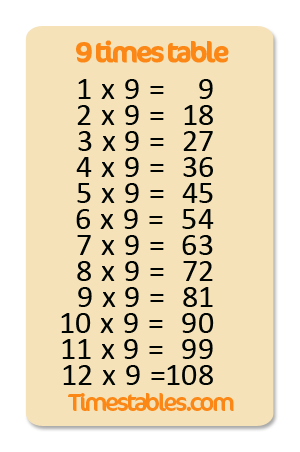# 9 times table

## The 5-step plan

• 9 x 1 = 9
• 9 x 2 = 18
• 9 x 3 = 27
• 9 x 4 = 36
• 9 x 5 = 45
• 9 x 6 = 54
• 9 x 7 = 63
• 9 x 8 = 72
• 9 x 9 = 81
• 9 x 10 = 90
• 9 x 11 = 99
• 9 x 12 = 108

### Step 1a: View, read aloud and repeat

Step 1a is to get familiar with the table, so view, read aloud and repeat. If you think you remember them it's time to test your knowledge at step 1b.

### Step 1b: In sequence

Fill in your answers. Once you have entered all the answers, click on 'Check' to see whether you have got them all right! If you got all the answers right, practice the 9 times table shuffled in random order.

### Step 3: Shuffled

Practice the 9 times table shuffled. Fill in all answers and press 'check' to see how many you got right.

### Step 4: Multiple choice

Try to answer all the 15 questions right!

### Step 5: Tables Diploma

Answer all the 24 questions right to get the diploma!

### Games

These games give the possibility to repeat the questions and improve the knowledge of the 9 multiplication table. Enjoy the 9 times table games!

## 9 times table memory

Try to find as fast a possible the matching questions and answers!

2
2 x 1
2
2 x 1
2
2 x 1
2
2
2
2
2
2
2
2
2
2

## 9 times table chart

This is where you can practice your 9 times table. You can practice the 9 times table in sequence and once you have got the hang of that you can make it a bit harder by practicing the sums up in random order. If you want to practice the 9 times table against the clock, you can of course take the speed test. If you want to practice at your leisure, we suggest you print out the 9 times table worksheet and practice with that. The 9 times table is a tricky table. What often helps is to use the tables you know well for answering the sums in the harder tables. One example of this is 6 x 9, which is often said to be tricky. If you do 5 x 9 first and then add 1 x 9 it is suddenly easier to answer. You can do this the other way round too. With 4 x 9 you can do 5 x 9 first then subtract 1 x 9. The nine times table is the multiplication table of 9 where we get the product of multiplying 9 with whole numbers. It is helpful to know the multiples of 9. Below you will see the 9 multiplication chart.What are all the 9 times tables?
The 9 times tables are:
• 9 x 1 = 9
• 9 x 2 = 18
• 9 x 3 = 27
• 9 x 4 = 36
• 9 x 5 = 45
• 9 x 6 = 54
• 9 x 7 = 63
• 9 x 8 = 72
• 9 x 9 = 81
• 9 x 10 = 90
• 9 x 11 = 99
• 9 x 12 = 108
What is the 9 times table pattern?
There is an interesting pattern in the 9 multiplication table. When you look at the 9 times table chart the digits in the tens column go from 0 to 9 and in the one’s column from 9 to 0. Look good at the 9 multiplication table chart, when you see the pattern it is easy to use.

## Print 9 times table worksheet

Click on the worksheet to view it in a larger format. For the 9 times table worksheet you can choose between three different sorts of exercise. In the first exercise you have to draw a line from the sum to the correct answer. In the second exercise you have to enter the missing number to complete the sum correctly. In the third exercise you have to answer the sums which have been shuffled.

## Description of the 9 times table

This is where you can practice the 9 times table by entering all the answers and then checking how many you got right. There are various ways the tables can be practiced. You are now on the 9 times table page where multiplication calculations can be practiced in sequence. Once you have mastered the table in sequence you can practice the sums in random order. You can learn the tables on a PC, tablet, iPad or mobile phone. You learn this table in year 4 maths.